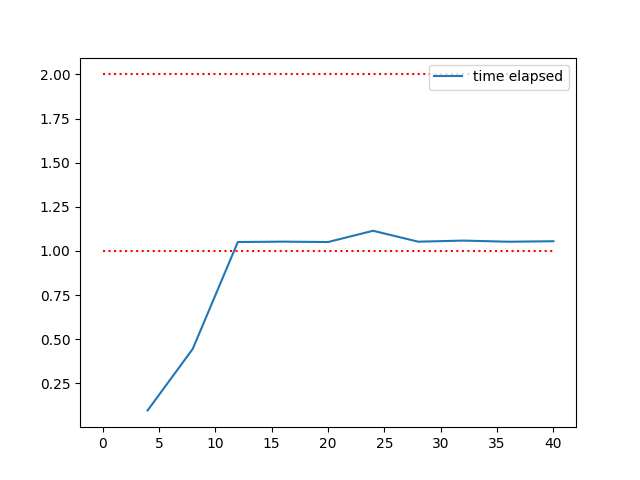# Plotting elapsed time in a meta-feature extraction

In this example, we will show you how the default value max_attr_num of meta-feature attr_conc was defined based on the total elapsed time of Iris dataset.```0. Number of attributes: 4 ...
1. Number of attributes: 8 ...
2. Number of attributes: 12 ...
3. Number of attributes: 16 ...
4. Number of attributes: 20 ...
5. Number of attributes: 24 ...
6. Number of attributes: 28 ...
7. Number of attributes: 32 ...
8. Number of attributes: 36 ...
9. Number of attributes: 40 ...
```

```# Load a dataset
import numpy as np
import pymfe.mfe
import matplotlib.pyplot as plt

# Added a default value for `max_attr_num` parameter of the `attr_conc`
# meta-feature extraction method, which is the most expensive meta-feature
# extraction method by far.

# The default parameter was determined by a simple inspection at the feature
# extraction time growing rate to the number of attributes on the fitted data.
# The threshold accepted for the time extraction is a value less than 2
# seconds.

# The test dataset was the iris dataset. The test code used is reproduced
# below.
np.random.seed(0)

arrsize = np.zeros(10)
time = np.zeros(10)

X = np.empty((iris.target.size, 0))

for i in np.arange(10):
X = np.hstack((X, iris.data))
print(f"{i}. Number of attributes: {X.shape} ...")
model = pymfe.mfe.MFE(features="attr_conc",
summary="mean",
measure_time="total").fit(X)
res = model.extract(suppress_warnings=True)

arrsize[i] = model._custom_args_ft["C"].shape
time[i] = res

plt.plot(arrsize, time, label="time elapsed")
plt.hlines(y=np.arange(1, 1 + int(np.ceil(np.max(time)))),
xmin=0,
xmax=arrsize[-1],
linestyle="dotted",
color="red")
plt.legend()
plt.show()

# The time cost of extraction for the attr_conc meta-feature does not grow
# significantly with the number of instance and, hence, it is not necessary to
# sample in the instance axis.
```

Total running time of the script: ( 0 minutes 9.215 seconds)

Gallery generated by Sphinx-Gallery